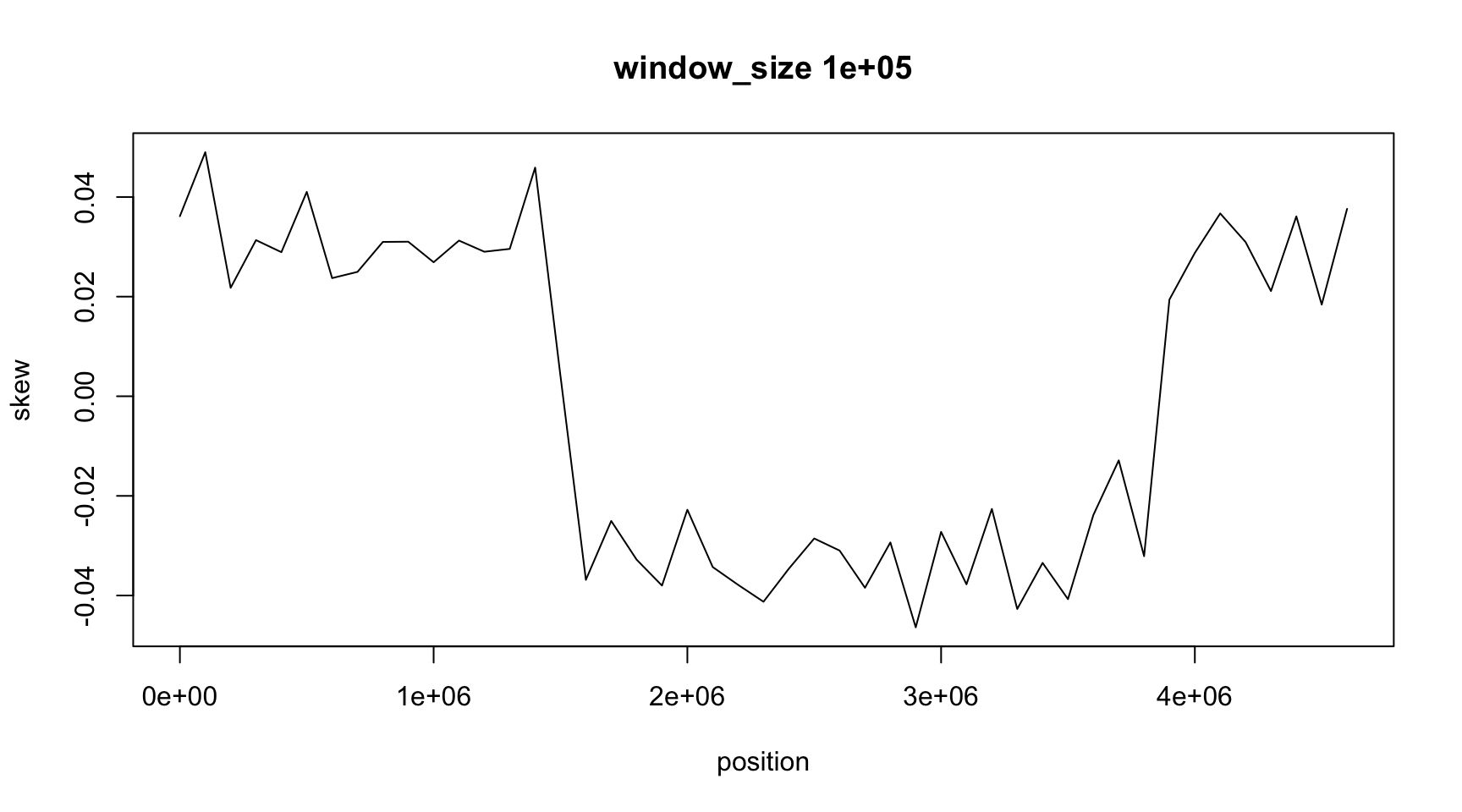March 14, 2018

## DNA has two strands

FASTA files show only one strand

We call it the forward strand

The other strand is not shown, but can be calculated

We call it the reverse strand

## Calculating the reverse strand

According to Watson & Crick, the reverse strand is complementary

• Change a by t and vice-versa
• Change c by g and vice-versa

Moreover, the reverse strand is reversed

• 5’ becomes 3’ and vice-versa
• i.e. we have to read it backwards
opposite_strand <- function(dna) {
ans <- rep(NA, lenght(dna))
for(i in 1:lenght(ans)) {
j <- lenght(dna) - i + 1
if(dna[j]=='a') {
ans[i] <- 't'
} else if(dna[j]=='c') {
ans[i] <- 'g'
} else if(dna[j]=='g') {
ans[i] <- 'c'
} else if(dna[j]=='t') {
ans[i] <- 'a'
}
}
return(ans)
}

## Another solution

Remember that we can give names to the elements of a vector

and we ca use the name as index

opposite_strand <- function(dna) {
ans <- rep(NA, lenght(dna))
compl <- c(a='t', c='g', g='c', t='a')
for(i in 1:lenght(ans)) {
j <- lenght(dna) - i + 1
ans[i] <- compl[ dna[j] ]
}
return(ans)
}

## What happens if we use the reverse strand

Will GC content change?

Will GC skew change?

## Local DNA statistics

In the previous class we calculated GC content and GC skew for the complete genome

Now we want to calculate them in regions of the genome

This way we can see if there are differences in DNA composition

These differences can have biological importance

## How to calculate values on regions

We will calculate the same formula on several DNA regions

Since it is the same pattern, we will prepare a function

What should be

• the output?
• the input(s)?
• the name?

## Defining a GC skew function

Remember that an R function is defined like this

name <- function(input1, input2, ...) {
Calculate
return(output)
}

## The inputs

The GC skew result should depend on:

• the genomic sequence
• the start of the region
• the length of the region

These are the parameters of the function. Do they have

• a name?
• a default value?

Note: avoid names that are already used for R functions

## The output

Here we have two cases

• For gc_content() the output is a number with the value $\frac{G+C}{A+T+G+C}$

• For gc_skew() the output is a number with the value $\frac{G-C}{G+C}$

In both cases the values in the formula are the number of nucleotides on the region

## The steps from input to output

How do we transform the input parameters into the output value?

We can use any R function available, such as

seq(from, to, by, length.out)

Note: each input of seq() is optional

## A possible solution

gc_content <- function(dna) {
freq <- table(dna)
ans <- (freq["g"]+freq["c"])/length(dna)
return(ans)
}

New Version:

gc_content <- function(dna, start=1, size=length(dna)) {
freq <- table(dna[seq(from=start, length.out=size)])
ans <- (freq["g"]+freq["c"])/size
return(ans)
}

Can you do the same for gc_skew()?

## Applying the function to many values

We want to evaluate gc_content() and gc_skew() on different positions of the genome

We traverse the genome with non-overlapping windows of fixed size

position <- seq(from=1, to=length(dna), by=window_size)

How do we apply gc_skew() to each element of position?

## One sample solution

position <- seq(from=1, to=length(dna), by=window_size)
for(i  in 1:length(position)) {
skew[i] <- gc_skew(dna, position[i], window_size)
}

What is wrong here?

## We cannot use [] if the vector does not exist

The first time we say skew[i] the vector skew does not exist

It has to be created before used

We can create it with just

skew <- NA

It is better if we declare the size

skew <- rep(NA, lenght(position))

## Complete code to evaluate local GC skew

window_size <- 1E5
position <- seq(from=1, to=length(dna), by=window_size)
skew <- rep(NA, length(position))
for(i  in 1:length(position)) {
skew[i] <- gc_skew(dna, position[i], window_size)
}

## Result

plot(position, skew, type="l",
main=paste("window_size", window_size))## The result depends on window_size

Can you calculate the local G skew for different window sizes?

Are they equal or different?

Can you calculate local GC content?

Is local GC content different in different parts of DNA?

## Result## Result## Result## Result## Finding the origin

As we discussed on the last class,

• the GC skew is positive in one part of chromosome
• and negative on the other, on average

The reason for this asymmetry is the DNA replication process

The origin of replication is in one of the sign changing places

## How can we find the change of sign

We want to write a program so we can find Ori on many different genomes

• Are both replichores of the same size?
• If they are different, what is the biological reason?
• Is there some insertion?
• What are the extra genes? What do they do?

## Finding the change of sign is not so easy

The values can change a lot between a window and the next one

Can you plot the GC-skew of each window versus the next window?

each point is (skew[i],skew[i+1])

## There is an easier way to find Ori

skew data is noisy. A lot of small random changes

Instead of looking directly at skew, we can look at cumulative skew

$\text{cum_skew}[i]=\sum_{j=1}^i \text{skew}[j]$

Now, instead of the change of sign, we look for the minimum and maximum (Why?)

How can we calculate cum_skew?

## Cumulative sum

It is easy to see that

$\text{cum_skew}[i]=\text{cum_skew}[i-1] + \text{skew}[i]$

So we can write something like this:

cum_skew <- skew
for(i in 2:length(skew)) {
cum_skew[i] <- cum_skew[i-1] + skew[i]
}

Can you write this as a function?

## Max & Min show the change of sign## Locating origin depends on window size

max(cum_skew)
 4.9877
which.max(cum_skew)
 155
position[which.max(cum_skew)]
 1540001

Ori is located between position[which.max(cum_skew)] and position[which.max(cum_skew)] + window_size

## How small can window_size be?

We can make the window very small

How would be skew and cum_skew if window_size=1